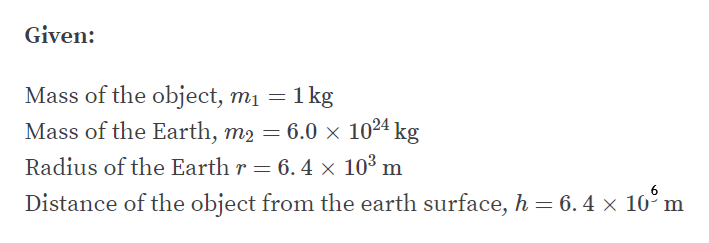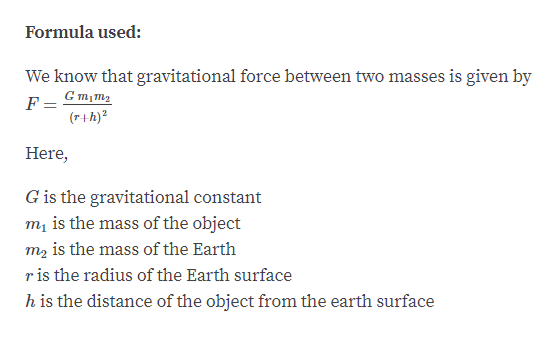# Calculate the force of gravity on same 1-kg mass if it were 6.4*106m above Earth's surface?

Question
2 views

Calculate the force of gravity on same 1-kg mass if it were 6.4*106m above Earth's surface?

check_circle

Step 1help_outlineImage TranscriptioncloseGiven: Mass of the object, m1 = 1 kg Mass of the Earth, m2 = 6.0 × 1024 kg Radius of the Earth r = 6. 4 × 10³ m Distance of the object from the earth surface, h= 6.4 × 10° m fullscreen
Step 2help_outlineImage TranscriptioncloseFormula used: We know that gravitational force between two masses is given by G m1m2 (r+h)? Here, G is the gravitational constant mų is the mass of the object m2 is the mass of the Earth ris the radius of the Earth surface h is the distance of the object from the earth surface fullscreen

### Want to see the full answer?

See Solution

#### Want to see this answer and more?

Solutions are written by subject experts who are available 24/7. Questions are typically answered within 1 hour.*

See Solution
*Response times may vary by subject and question.
Tagged in

### Physics